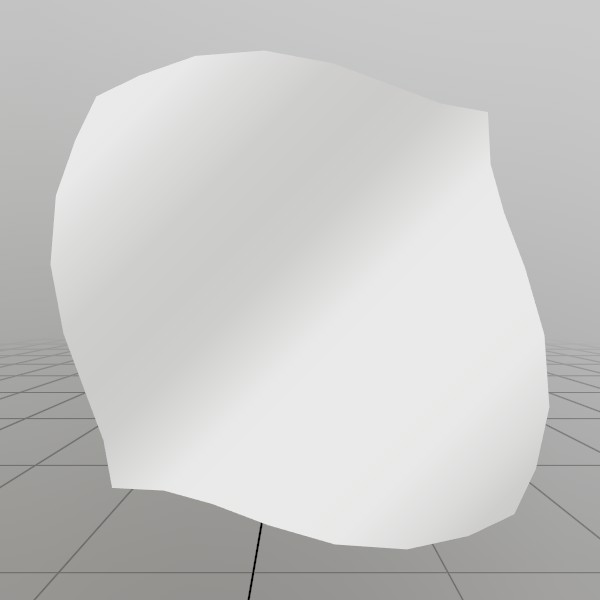# Mesh.SetData

``````void SetData(Vertex[] vertices, UInt32[] indices, bool calculateBounds)
``````

Assigns the vertices and indices for this Mesh! This will create a vertex buffer and index buffer object on the graphics card. If you’re calling this a second time, the buffers will be marked as dynamic and re-allocated. If you’re calling this a third time, the buffer will only re-allocate if the buffer is too small, otherwise it just copies in the data!

Remember to set all the relevant values! Your material will often show black if the Normals or Colors are left at their default values.

Calling SetData is slightly more efficient than calling SetVerts and SetInds separately.

Vertex[] vertices An array of vertices to add to the mesh. Remember to set all the relevant values! Your material will often show black if the Normals or Colors are left at their default values.
UInt32[] indices A list of face indices, must be a multiple of 3. Each index represents a vertex from the provided vertex array.
bool calculateBounds If true, this will also update the Mesh’s bounds based on the vertices provided. Since this does require iterating through all the verts with some logic, there is performance cost to doing this. If you’re updating a mesh frequently or need all the performance you can get, setting this to false is a nice way to gain some speed!

## Examples

### Procedurally generating a wavy gridHere, we’ll generate a grid mesh using Mesh.SetVerts and Mesh.SetInds! This is a common example of creating a grid using code, we’re using a sin wave to make it more visually interesting, but you could also substitute this for something like sampling a heightmap, or a more interesting mathematical formula!

Note: x+y*gridSize is the formula for 2D (x,y) access of a 1D array that represents a grid.

``````const int   gridSize = 8;
const float gridMaxF = gridSize-1;
Vertex[] verts = new Vertex[gridSize*gridSize];
uint  [] inds  = new uint  [gridSize*gridSize*6];

for (int y = 0; y < gridSize; y++) {
for (int x = 0; x < gridSize; x++) {
// Create a vertex on a grid, centered about the origin. The dimensions extends
// from -0.5 to +0.5 on the X and Z axes. The Y value is then sampled from
// a sin wave using the x and y values.
//
// The normal of the vertex is then calculated from the derivative of the Y
// value!
verts[x+y*gridSize] = new Vertex(
new Vec3(
x/gridMaxF-0.5f,
SKMath.Sin((x+y) * 0.7f)*0.1f,
y/gridMaxF-0.5f),
new Vec3(
-SKMath.Cos((x+y) * 0.7f),
1,
-SKMath.Cos((x+y) * 0.7f)).Normalized,
new Vec2(
x / gridMaxF,
y / gridMaxF));

// Create triangle face indices from the current vertex, and the vertices
// on the next row and column! Since there is no 'next' row and column on
// the last row and column, we guard this with a check for size-1.
if (x<gridSize-1 && y<gridSize-1)
{
int ind = (x+y*gridSize)*6;
inds[ind  ] = (uint)((x+1)+(y+1)*gridSize);
inds[ind+1] = (uint)((x+1)+(y  )*gridSize);
inds[ind+2] = (uint)((x  )+(y+1)*gridSize);

inds[ind+3] = (uint)((x  )+(y+1)*gridSize);
inds[ind+4] = (uint)((x+1)+(y  )*gridSize);
inds[ind+5] = (uint)((x  )+(y  )*gridSize);
}
} }
demoProcMesh = new Mesh();
demoProcMesh.SetData(verts, inds);
``````

Found an issue with these docs, or have some additional questions? Create an Issue on Github!